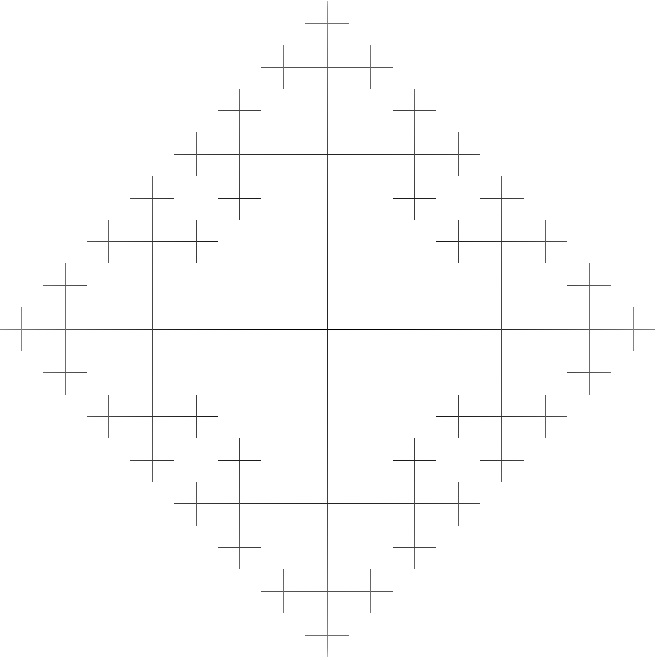By Adrian Hendrawan Putra, The University of Melbourne

I was first introduced to mathematics through graph theory. Graphs is used in computer science to model certain computational problems and graph algorithms are one of the central focus of our computer science classes. Initially I was planning on majoring in computer science, but I became interested in mathematics when I realised I enjoyed proving the correctness of graphs algorithms a lot more than I do implementing them. It was then when I decided to give mathematics a try. Initially, I had promised to myself to stay true to my background and focus on the areas of mathematics that are relevant to computer science, such as combinatorics and graph theory. Not long after, I came across group theory and broke this promise.

One of the most appealing quality of mathematics as a subject is its interconnectedness. A connection between graphs and groups is provided by covering space theory in algebraic topology. To put it simply, a fundamental group of a space is the set of loops on said space, up to continuous deformation. If we imagine the figure eight (i.e. “8”) we see that there are two loops making the figure eight. Lets call the loop on the top of the figure eight the “a” loop while the loop on the bottom the “b” loop. It should not be surprising thus, that the fundamental group of the figure eight space is given by the free group on two generators “a” and “b”. Explicitly, this means that elements of this group are given by words made of “a” and “b” (and their inverses) and we can draw a graph corresponding to these words. The image included is the Cayley graph of the free group on the two generators, and it is also the universal covering space of the figure eight space.Adrian Hendrawan Putra was a recipient of a 2018/19 AMSI Vacation Research Scholarship.